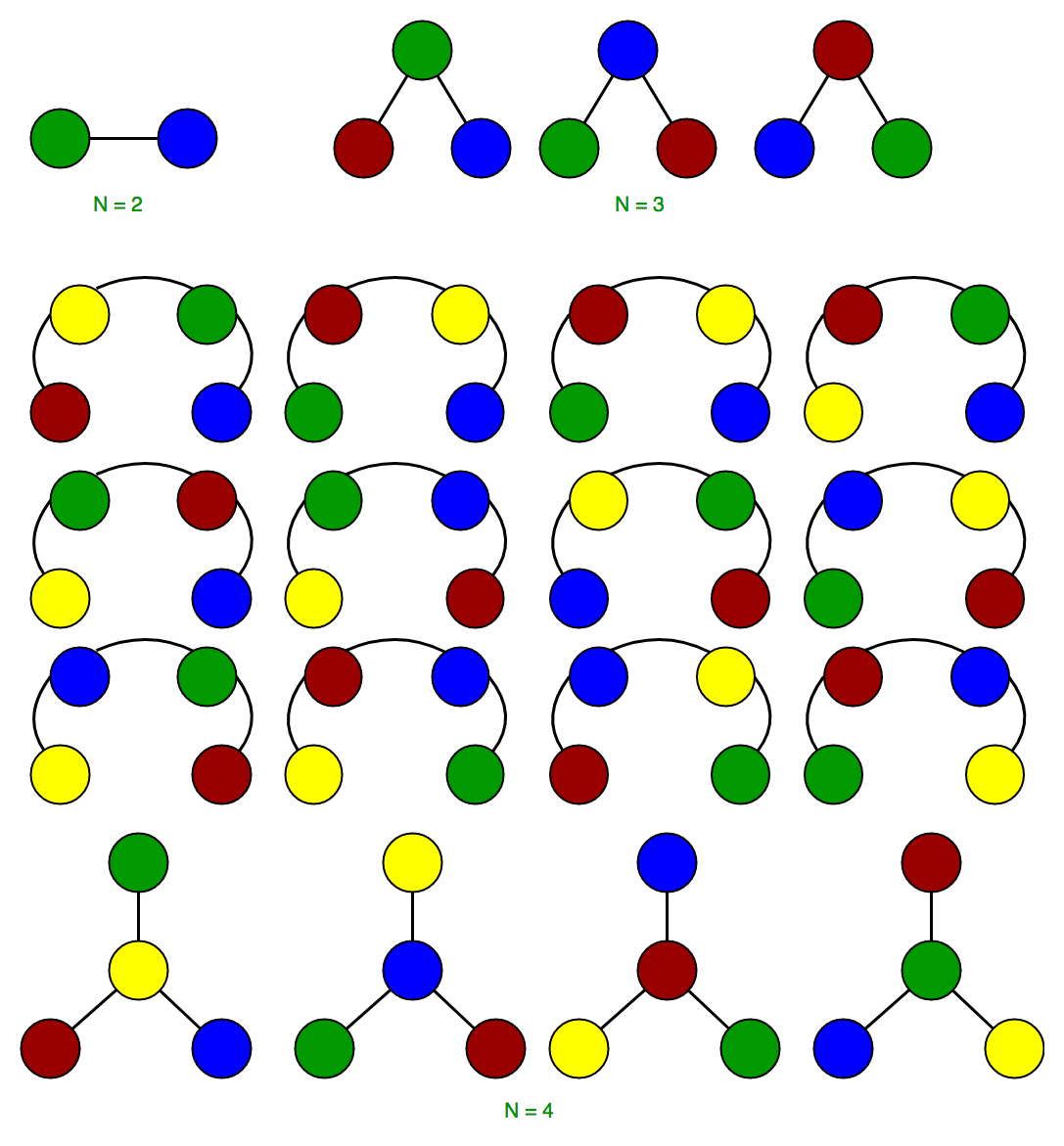# G-Fact 20 (Cayley’s formula for Number of Labelled Trees)

• Difficulty Level : Easy
• Last Updated : 13 Feb, 2018

Consider below questions.

1. How many spanning trees can be there in a complete graph with n vertices?
2. How many labelled Trees (please note trees, not binary trees) can be there with n vertices?

The answer is same for both questions.

For n = 2, there is 1 tree.

For n = 3, there are 3 trees.

For n = 4, there are 16 treesThe formula states that for a positive integer n, the number of trees on n labeled vertices is nn-2